## Example Questions

### Example Question #1 : How To Find The Length Of The Diagonal Of A Quadrilateral

A square has a length of.  What must be the length of the diagonal?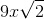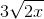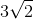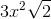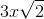Explanation:

A square with a length ofindicates that all sides aresince a square has 4 equal sides.  Use the Pythagorean Theorem to solve for the diagonal.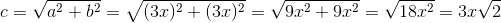### Example Question #2 : How To Find The Length Of The Diagonal Of A Quadrilateral

If the rectangle has side lengths of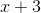and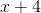, what is the diagonal of the rectangle?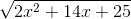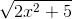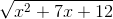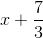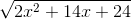Explanation:

Use the Pythagorean Theorem to solve for the diagonal.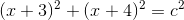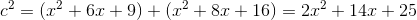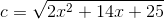### Example Question #3 : How To Find The Length Of The Diagonal Of A Quadrilateral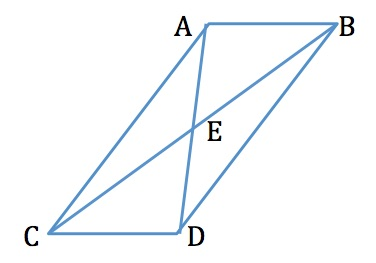In the parallelogram above,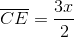and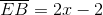. What is the length of the diagonal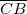?Explanation:

In a parallelogram, the two diagonals bisect each other at their centers. Therefore,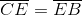. To find, we set them equal to one another and solve.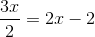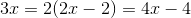Plugging this value back into both equations, then adding those values together, gives us our answer of